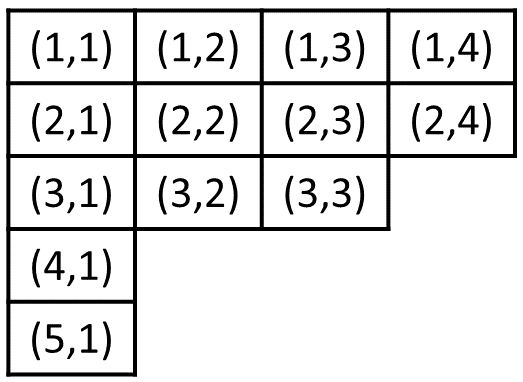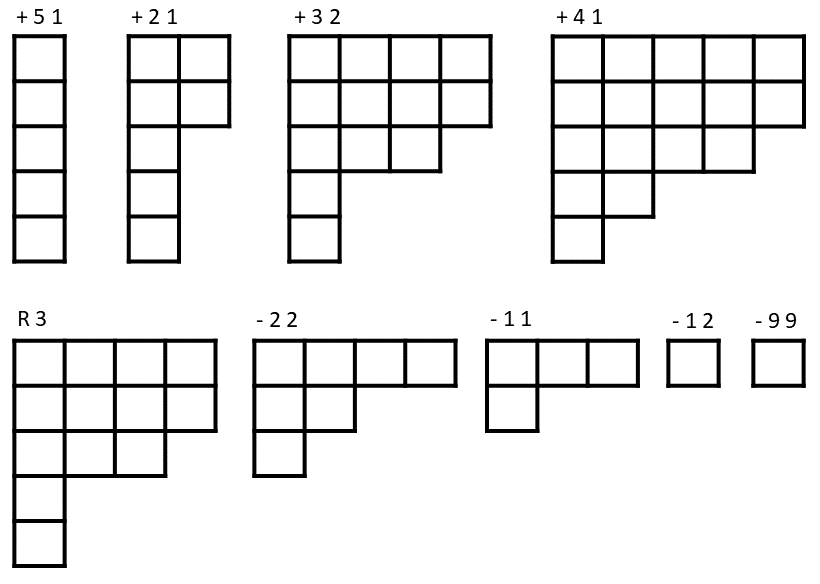# #789. 【CTS2023/WC2023】楼梯

### 题目描述

• 若 $(x,y)\in L$ 且 $x>1$，则 $(x-1,y)\in L$。
• 若 $(x,y)\in L$ 且 $y>1$，则 $(x,y-1)\in L$。

$$\{(a-x+1,b-y+1)\mid(a,b)\in L,a\ge x,b\ge y\}.$$• 给定正整数 $a$ 和 $b$，在前 $a$ 行的末尾插入 $b$ 格。形式化地，对于 $i=1,2,\cdots,a$，将 $(i,s_i+1),(i,s_i+2),\cdots,(i,s_i+b)$ 加入 $L$。
• 给定正整数 $a$ 和 $b$，在第 $a$ 行后（包含第 $a$ 行）的所有行行末尾删去 $b$ 格，若不足则删空。形式化地，对于 $i=a,a+1,a+2,\cdots,10^{100}$，将 $(i,s_i),(i,s_i−1),\cdots,(i,s_i−b+1)$ 从 $L$ 中移除（不存在的则忽略）。
• 给定正整数 $u$，撤销之前的 $u$ 次操作，即将楼梯还原为 $u$ 次操作前的状态，保证这 $u$ 次操作均为询问或在行末尾插入。具体地，假设该操作为第 $t$ 次操作，我们一定有 $t>u$，且第 $t−1,t−2,\cdots,t−u$ 次操作均为询问或在行末尾插入（即上述的第一种修改）。你只需要将楼梯还原为第 $t−u$ 次操作前的状态即可（当然，你应该保留询问的输出）。

### 输入格式

• + a b：在前 $a$ 行的末尾插入 $b$ 格。
• - a b：在第 $a$ 行后（包括第 $a$ 行）的所有行行末尾删去 $b$ 格，若不足则删空。
• R u：撤销之前的 $u$ 次操作，即将楼梯还原为 $u$ 次操作前的状态。保证这 $u$ 次操作存在且均为询问或在行末尾插入，即该行之前的 $u$ 行均以 +? 开头。
• ? q：询问是否有边界格数等于 $q$ 的子楼梯，若有则给出任意合法生成格。保证 $q$ 是当前楼梯边界格数的因子

### 样例一

#### input

18
+ 5 1
+ 2 1
? 3
+ 3 2
? 4
? 4
+ 4 1
? 3
R 3
- 2 2
? 3
- 1 1
? 2
? 4
- 1 2
? 1
- 9 9
? 1

#### output

3 1
1 3
2 2
2 4
2 1
1 2
1 1
1 1
1 1

#### explanation### 子任务

• 对于 +- 操作，$1\le a,b\le10^9$。
• 对于 R 操作，保证之前紧邻的 $u$ 次操作存在且均为询问或在行末尾插入。
• 对于 ? 操作，$1\le q\le10^{18}$ 且保证为当前楼梯边界格数的因子